Function Repository Resource:

# PerfectPowerQ

Determine whether a given integer is a perfect power

Contributed by: Swastik Banerjee
 ResourceFunction["PerfectPowerQ"][n] yields True if n can be represented as a perfect power of a some integer greater than 1 and False otherwise. ResourceFunction["PerfectPowerQ"][n,k] yields True if n is the kth power of some integer greater than 1 where k≥2.

## Details and Options

An integer n is a perfect power if there exist integers x and k such that xk=n, where x,k2.
An integer has a perfect power if the set of exponents in its prime factorization have a GCD greater than one.

## Examples

### Basic Examples (1)

Test whether 100 can be expressed as a perfect power:

 In:=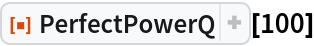Out=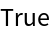### Scope (1)

List of all perfect powers up to 105:

 In:=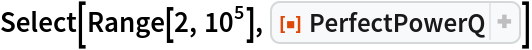Out=### Options (1)

When a second argument k is passed, PerfectPowerQ checks whether n can be represented as the perfect kth power of some integer greater than one:

 In:=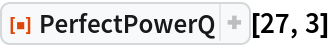Out=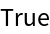### Applications (1)

The first few perfect powers without duplications are 1, 4, 8, 9, 16, 25, 27, 32, 36, 49, 64, 81, 100, 121, 125, … (OEIS A001597). Even more amazingly, the sum of the reciprocals of these numbers (excluding 1) is given by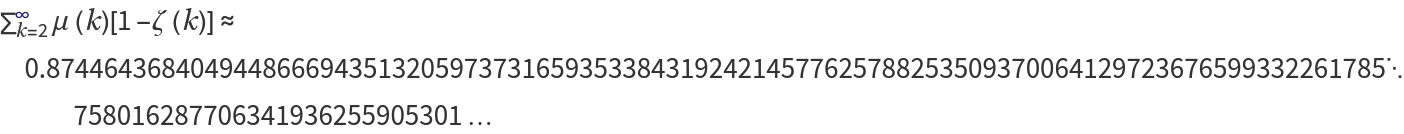(OEIS A072102), where μ(k) is the Möbius function (MoebiusMu) and ζ(k) is the Riemann zeta function (Zeta).

This can be verified with the PerfectPowerQ function:

 In:=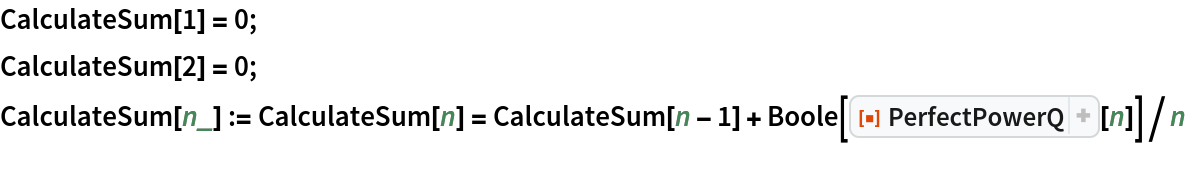In:=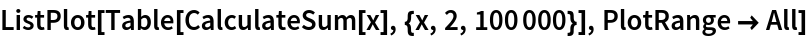Out=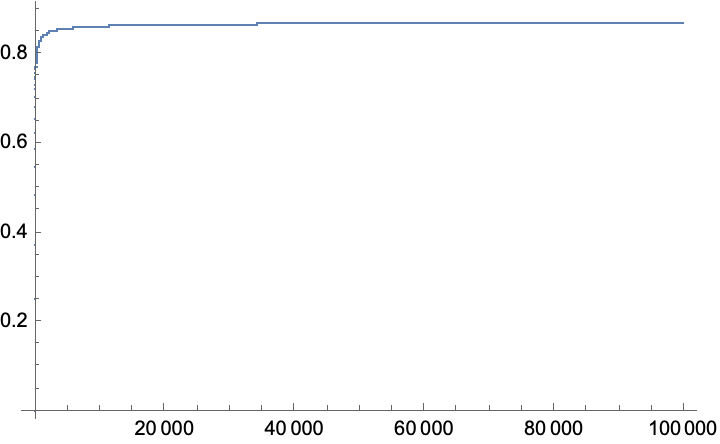### Possible Issues (2)

The first argument should be an integer greater than 1:

 In:=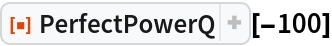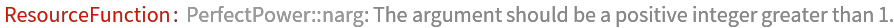Out=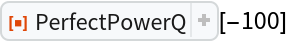The second argument, if given, should be greater than or equal to 2:

 In:=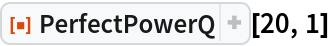Out=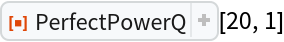### Properties and Relations (1)

PerfectPowerQ of a prime number always yields False:

 In:=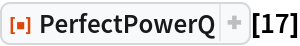Out=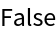## Publisher

Wolfram Summer School

## Version History

• 1.0.0 – 25 September 2020